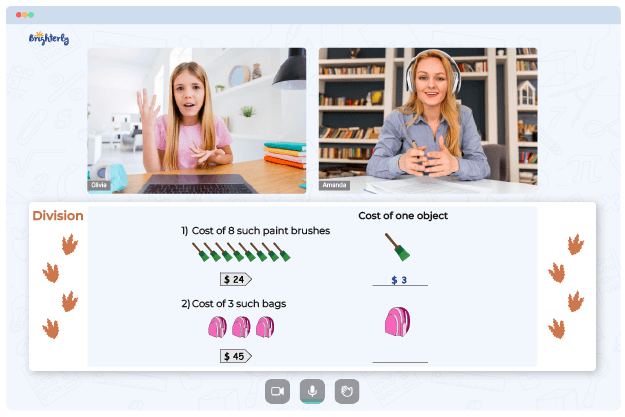# Greatest Common Factor Worksheets

Also called the greatest common divisor, the greatest common factor is an important math concept that comes in handy when solving fraction problems. These greatest common factor worksheets will help students find the greatest common factor of given numbers. Before downloading the greatest common factor worksheets PDF, let’s learn about GCR and its benefits.

## What is Greatest Common Factor

The greatest common factor is the highest factor that a set of numbers have in common. It can also be defined as the largest number divided into two or more numbers. To understand the concept of GCF better, you’ll need to understand what a factor is.

Factors are numbers that can divide into a given number completely (with no remainder.) However, a common factor is any number that other numbers have in common. Hence, the greatest common factor between two or more numbers is the highest number that is a factor or sets of numbers given (two or more.)

Math for Kids

Is Your Child Struggling With Math?
1:1 Online Math TutoringYou can find the greatest common factor by using the prime factorization method or listing all the factors.

## The greatest common factor worksheet Benefits

This factoring gcf worksheet will help students to understand the greatest common factor and learn how to find the greatest common factor between two or more numbers. Also, this is a factoring by gcf worksheet with answers. There are lots of examples that show how to solve the greatest common factor problems using different methods. Hence, students can study the problems and answers and learn how to solve GCF problems on their own.### Greatest Common Factor Worksheets PDF

Factoring GCF Worksheet### Greatest Common Factor Worksheets PDF

Greatest Common Factor Worksheet### Greatest Common Factor Worksheets PDF

Greatest Common Factor Worksheets PDF### Greatest Common Factor Worksheets PDF

Factoring With GCF Worksheet

Also, the gcf factoring worksheet will walk students through the concept of the greatest common factor, like a step-by-step guide. Visual illustrations are provided to make the learning process smooth and easy to follow. Whether your child is struggling with factoring by GCF or already good at it, this factoring with gcf worksheet was created to help them get even better.

### More Worksheets

Need help with Division?• Does your child struggle to grasp the division of math?
• Try lessons with an online tutor.

Is your child having trouble understanding division? An online tutor could provide the necessary support.